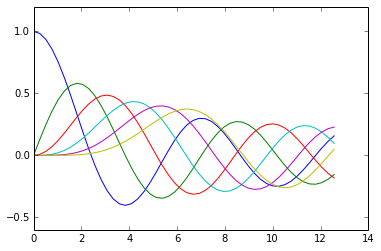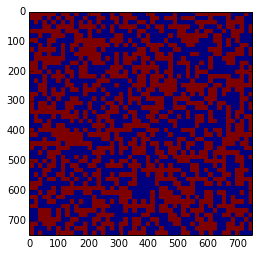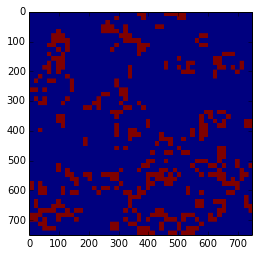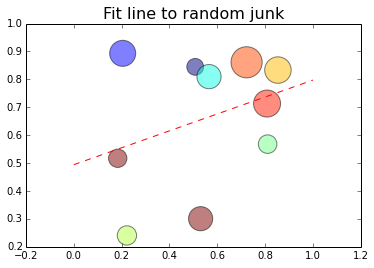# How to Python Like a Boss¶

## Anaconda & Scipy for data analysis¶GitHub Source

# In short:¶

1. Use a Python distribution
2. Use IPython + notebook
3. Use the Scipy stack

# But why Python?¶

• It's the best thing ever

# No, seriously¶

• Expressive language with easy-to-read syntax makes it easier to share and reuse code
• Large ecosystem of high-quality modules makes it easy to not reinvent the wheel

# 1. Use a Python Distribution¶

No, scratch that...

# 1. Use Anaconda Python Distribution¶

### Convenience¶

• Many popular packages for science and data analysis preinstalled

### Portability¶

• The Simpsons (CNetS)
• FutureGrid
• Big Red II
• Default Python installs on these machines are out of date

### Compatibility¶

• Fix the "Python Packaging Problem" with dependency resolution
• Anaconda uses conda as its package manager, instead of pip or easy_install
• Use separate environments for incompatible packages

### Shareability¶

• Freeze the requirements for a particular package or script
• Share the requirements as dependencies
• If using Anaconda, share the entire environment

# DEMOS¶

• Installing and updating Anaconda
• Installing packages with conda and pip
• Using conda for package environments

Docs can be found online.

# Installing and updating Anaconda¶

This works on campus machines

    wget <copy-paste that link>


Answer yes at the prompts. At the end, the installer asks if you want to add Anaconda to your PATH, say yes if this will be your primary Python install.

To update all of anaconda's packages at once:

conda update anaconda

# Installing packages with conda and pip¶

Conda is preferable since it does dependency resolution:

conda install pymc

But not all packages are in the conda repositories

conda install geopy



In that case, use pip

pip install geopy

# Using conda for package environments¶

The current version of NetworkX is 1.8.1, but suppose I have a script that is dependent on NetworkX 1.7 for now. This calls for package environments!

• Create a new environment named "nx1.7", and link all the Anaconda packages

  conda create -n nx1.7 anaconda
• List all currently-defined environments:

  conda info -e
• Activate our new environment:

  source activate nx1.7
• Replace NetworkX 1.8 with 1.7:

  conda install networkx=1.7
• Deactivate our new environment, returning to the base Anaconda env:

  source deactivate nx1.7

Environments are also a great way to have Python 2.7 and 3.3 side-by-side:

    conda create -n py3.3 python=3.3 anaconda



Then, like before, I can just switch to py3k with a

    source activate py3.3



and switch back with

    source deactivate py3.3

# 2. Use IPython¶# 2.1 IPython shell¶

• Enhanced Python shell
• Comes in console and Qt versions
• Awesome features
• Tab-completion
• Magic (special %-prefixed commands)
• Inline plots in Qt console

# IPython magic¶

In :
import random as rd
import numpy as np

In :
%timeit a = [rd.random() for x in range(1000)]

10000 loops, best of 3: 102 µs per loop

In :
%timeit a = [np.random.random() for x in range(1000)]

10000 loops, best of 3: 182 µs per loop

In :
%timeit a = np.random.random(1000)

100000 loops, best of 3: 14 µs per loop


There are also cell magics for use in IPython notebook

In :
%%time
# This is a terrible way to do this
fib = [0, 1]
for i in range(10**5):
fib.append(fib[-1] + fib[-2])

CPU times: user 208 ms, sys: 24 ms, total: 232 ms
Wall time: 210 ms


# DEMO Qt console¶

Run the following:

    ipython qtconsole --pylab inline


then paste this in the Qt console:

In :
from scipy.special import jn
x = linspace(0, 4*pi)
for i in range(6):
plot(x, jn(i, x))# 2.2 IPython notebook¶

Locally, you can run

    ipython notebook --pylab inline

• Evaluate and edit code by the chunk (cell) instead of by line
• Keep images/plots/calculations inline with code
• Excellent for interactive/iterative data exploration

# DEMO IPython Notebook¶

If you're reading this, you're seeing my slides online. It's hard for me to demo this for you, but luckily there are writeups and screencasts out there of how awesome IPython notebook is.# Bonus: NBconvert¶

• Converts notebooks into a variety of formats
• LaTeX/pdf
• HTML
• Reveal.js
• This entire presentation was made with IPython notebook! GitHub Source
• Notebooks can then be hosted/shared with NBviewier or Wakari

# Extra Bonus: Python + JavaScript in the notebook¶

Run this notebook in Wakari to see Python + JavaScript in actionActually...

# 3. Use the rest of the SciPy stack¶# 3.1 Numpy¶

• Used for n-dimensional arrays
• C code under the hood, so it's really fast when used correctly
• Use it to replace MATLAB/Octave

# Example¶

The "native" Python solution for matrices is often to use a list of lists, but this can be really awful.

For example, let's look at column-wise operations on a list of lists.

In :
import random as rd
N = 4

lol = [[rd.random() for c in range(N)] for r in range(N)]
# OR
lol = []
for r in range(N):
row = []
for c in range(N):
row.append(rd.random())
lol.append(row)
lol

Out:
[[0.9937229473980533,
0.995334868014186,
0.5942674962738761,
0.5154385022192677],
[0.422784005229769,
0.7807343114323023,
0.09179473422846407,
0.609573372880339],
[0.4972764651566518,
0.13311678867268917,
0.12249203373176598,
0.8453804747179231],
[0.15205034547325147,
0.6133030575174816,
0.9485418183964225,
0.8287048466130321]]

List slicing: Can we get the first column of this "matrix?"?

In :
lol[:,0]

---------------------------------------------------------------------------
TypeError                                 Traceback (most recent call last)
<ipython-input-55-fb5f0d5bbc73> in <module>()
----> 1 lol[:,0]

TypeError: list indices must be integers, not tuple

Try again.

In :
lol[:]

Out:
[0.9937229473980533, 0.995334868014186, 0.5942674962738761, 0.5154385022192677]

This is actually the first row. Seriously, go back and check.

Surely a list comprehension can save us:

In :
[x for x in lol]

Out:
[0.9937229473980533,
0.422784005229769,
0.4972764651566518,
0.15205034547325147]

That works, but... ugh.

Do you want to write a column-wise sum?

This isn't FORTRAN. I shouldn't have to think about which way my matrix is laid out, row- or column-major. Try again with Numpy:

In :
import numpy as np

npm = np.random.random((N, N))
npm

Out:
array([[ 0.15602394,  0.98540191,  0.13926817,  0.23745073],
[ 0.51627372,  0.53242708,  0.27257836,  0.30676598],
[ 0.04349275,  0.16361163,  0.3415986 ,  0.49103593],
[ 0.33081051,  0.99886844,  0.76993015,  0.55732118]])
In :
npm[:,0]

Out:
array([ 0.15602394,  0.51627372,  0.04349275,  0.33081051])

Nice. And that column-wise sum?

In :
npm.sum(0)

Out:
array([ 1.04660092,  2.68030907,  1.52337528,  1.59257382])

Like a boss.

# Bonus Example: Conway's Game of Life¶

Uses Numpy's image convolution for a really fast, really elegant Game of Life implementation.

In :
import numpy
import scipy.ndimage
import Image

class Life(object):

def __init__(self, n, p=0.5, mode='wrap'):
self.n = n
self.mode = mode
self.array = numpy.uint8(numpy.random.random((n, n)) < p)
self.weights = numpy.array([[1,1,1],
[1,10,1],
[1,1,1]], dtype=numpy.uint8)

def step(self):
con = scipy.ndimage.filters.convolve(self.array,
self.weights,
mode=self.mode)

boolean = (con==3) | (con==12) | (con==13)
self.array = numpy.int8(boolean)

def run(self, N):
for _ in range(N):
self.step()

def draw(self, scale):
im = Image.fromarray(numpy.uint8(self.array)*255)
z = int(scale*self.n)
return im.resize((z,z))

In :
l = Life(50)
imshow(l.draw(15))

Out:
<matplotlib.image.AxesImage at 0x547cf10>In :
l.run(10)
imshow(l.draw(15))

Out:
<matplotlib.image.AxesImage at 0x55d0210># 3.2 Scipy library¶

• Higher-level interface for numeric computation with Numpy
• Many submodules for common computational tasks
• scipy.stats
• scipy.optimize
• scipy.linalg
• scipy.signal
• scipy.sparse.csgraph (compressed sparse graph)
• and much more
• Use it to replace R and MATLAB/Octave

# Example: Linear Regression¶

In :
from scipy import stats
import numpy as np

x = np.random.random(10)
y = np.random.random(10)
slope, intercept, r_value, p_value, std_err = stats.linregress(x,y)

print "r-squared:", r_value**2

r-squared: 0.109819072488


We'll come back to this in a minute.

# 3.3 Matplotlib¶

• Comprehensive 2D plotting library
• Incredibly flexible and powerful
• Steep learning curve, similar to native MATLAB and R plotting

# Example: Linear Regression, continued¶

From before, we have the following:

In [ ]:
from scipy import stats
import numpy as np

x = np.random.random(10)
y = np.random.random(10)
slope, intercept, r_value, p_value, std_err = stats.linregress(x,y)

print "r-squared:", r_value**2

In :
import matplotlib.pyplot as plt

sizes = 1000* np.random.random(10)
colors = np.random.random(10)

fit_x = np.linspace(0,1,100)
fit_y = slope * xx + intercept

plt.scatter(x,y, sizes, colors, alpha=0.5)
plt.plot(fit_x, fit_y, '--r')

plt.title("Fit line to random junk", fontsize=16)
plt.show()# 3.4 Pandas¶

• Used for manipulating tabular data
• SQL query output
• CSV
• Has many features for time series
• Allows very expressive syntax
• Provides a Data Frame structure similar to that in R
• Has NumPy at the core, so can be very fast

# Example: Kinsey Reporter's timelines¶

TASK: Calculate how many responses Kinsey Reporter received, with weekly resolution, for a given tag.

• Reports come in, and each report has one or more tags associated with it.
• First, we need to do a SQL query to get all the reports associated with a specific tag (don't worry if you're not familiar with SQL)

  SELECT option,
DATE(timestamp) as datestamp,
WHERE event_id = survey_event.id
AND option_id = survey_option.id
GROUP BY datestamp

The results come to Python looking like this:

[
...
('smile flirt', datetime.date(2013,5,23), 12),
('smile flirt', datetime.date(2013,5,24), 9),
...
]

To calculate the weekly timeline with native Python would be... uncomfortable. One must:

1. Fill in any missing days with zeros
2. Sort the list by date, go through and sum up every set of seven counts

With Pandas, it's as simple as:

import pandas as pd

df = pd.DataFrame.from_records(kr_rows, index=datestamp)
timeline = df['num_answers'].asfreq('D').resample('W', how=sum).fillna(0)

# Example: Auto MPG analysis¶

In addition to making time series easier, Pandas makes plotting a snap too.

This is a notebook where I slice and dice some automobile gas mileage data: https://www.wakari.io/sharing/bundle/clayadavis/mpg

Key concepts used in the MPG analysis:

• DataFrame.group_by()
• Series.describe()
• Series.plot()
• DataFrame.boxplot()
• DataFrame.hist()
• Series.rolling_mean()

# 3.5 Sympy¶

• Symbolic math library
• Do all that algebra/calculus you're terrible at!
• Simplification
• Expansion
• Integration
• Differentiation
• etc.
• If output supports it (e.g. IPython notebook), prints LaTeX or unicode output
• Use it to replace Mathematica

# Example: Differentiation¶

In :
import sympy
sympy.init_printing(use_latex=True) ##or use_unicode=True in a console
x, y = sympy.symbols('x y')


We differentiate and get an answer...

In :
sympy.diff(sympy.exp(x**2), x)

Out:
$$2 x e^{x^{2}}$$

...or we can create an unevaluated expression for further manipulation...

In :
my_deriv = sympy.Derivative(sympy.exp(x**2), x, x)
my_deriv

Out:
$$\frac{d^{2}}{d x^{2}} e^{x^{2}}$$

...which we can evaluate later.

In :
my_deriv.doit()

Out:
$$2 \left(2 x^{2} + 1\right) e^{x^{2}}$$

# More Sympy examples¶

In :
sympy.Integral(sympy.exp(-x**2 - y**2), x, y)

Out:
$$\iint e^{- x^{2} - y^{2}}\, dx\, dy$$
In :
from sympy import oo
sympy.integrate(sympy.exp(-x**2 - y**2), (x, -oo, oo), (y, -oo, oo))

Out:
$$\pi$$
In :
sympy.solve([x*y - 7, x + y - 6], [x, y])

Out:
$$\begin{bmatrix}\begin{pmatrix}- \sqrt{2} + 3, & \sqrt{2} + 3\end{pmatrix}, & \begin{pmatrix}\sqrt{2} + 3, & - \sqrt{2} + 3\end{pmatrix}\end{bmatrix}$$

# OMFG Example: Sympy Gamma¶

• Use it to replace Wolfram Alpha PRO# 3.x Honorable SciPy mention: scikit-learn¶

Mature and featureful library for machine learning. Link# Fin¶

Thanks for your time. Hit me up on Twitter or email if you have any questions.

# 4. Make web GUIs¶

• As a community, we've focused on creating awesome tools for data analysis
• Less attention has been paid to sharing workflows once created
• IPython notebook is a huge step in the right direction
• GUIs are cool, they lower the barrier of entry

• GUIs suck to program
• For 90% of use cases, a web GUI on a modern browser is as good as native
• 90% of (data) scientists want the same thing from a GUI:
• Give me knobs to twiddle
• Let me see how it affects the output

# Enter Ashiba¶

• A framework for making webapps with Python at their core
• GUI elements defined in HTML or Enaml
• No JavaScript needed
• (almost) free software
• Backed by Continuum Analytics

Why it rocks:

• Uses familiar Python libraries on the backend
• Pandas
• Numpy
• Matplotlib
• Allows rapid development of web applications from existing Python code
• Moves beyond "open data" -- share both the data and a framework to analyze it
• Useful at every stage of te research process
• Exploring data and forming hypotheses
• Eliciting collaboration and feedback from peers
• Enabling wide dialog and evaluation of the finished product

# DEMOS¶# 5. Profile and Compile¶

Rule 1: "Premature optimization is the root of all evil" $-$ Knuth

Rule 2: Post-hoc optimization is fucking rad